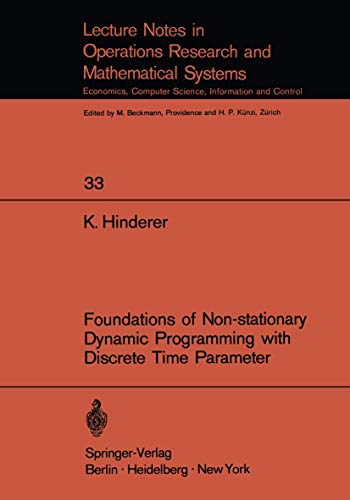Free Shipping in Australia
Proud to be B-Corp

# Foundations of Non-stationary Dynamic Programming with Discrete Time Parameter K. Hinderer\$194.69
Condition - New
Only 2 left

## Summary

dynamic programming under risk, dynamic programming under uncertainty, Markovian models, stationary models, and models with finite horizon from a unified point of view.

## Foundations of Non-stationary Dynamic Programming with Discrete Time Parameter Summary

### Foundations of Non-stationary Dynamic Programming with Discrete Time Parameter by K. Hinderer

The present work is an extended version of a manuscript of a course which the author taught at the University of Hamburg during summer 1969. The main purpose has been to give a rigorous foundation of stochastic dynamic programming in a manner which makes the theory easily applicable to many different practical problems. We mention the following features which should serve our purpose. a) The theory is built up for non-stationary models, thus making it possible to treat e.g. dynamic programming under risk, dynamic programming under uncertainty, Markovian models, stationary models, and models with finite horizon from a unified point of view. b) We use that notion of optimality (p-optimality) which seems to be most appropriate for practical purposes. c) Since we restrict ourselves to the foundations, we did not include practical problems and ways to their numerical solution, but we give (cf.section 8) a number of problems which show the diversity of structures accessible to non stationary dynamic programming. The main sources were the papers of Blackwell (65), Strauch (66) and Maitra (68) on stationary models with general state and action spaces and the papers of Dynkin (65), Hinderer (67) and Sirjaev (67) on non-stationary models. A number of results should be new, whereas most theorems constitute extensions (usually from stationary models to non-stationary models) or analogues to known results.

1. Introduction and summary.- I. Countable state space.- 2. Decision models and definition of the problem.- 3. The principle of optimality and the optimality equation.- 4. Value iteration.- 5. Criteria of optimality and existence of $$\\bar{p}$$-optimal plans.- 6. Sufficient statistics, Markovian and stationary models.- 7. Models with incomplete information.- 8. Examples of special models.- 9. Randomized plans.- 10. Dynamic programming under uncertainty.- II. General state space.- 11. Decision models.- 12. Measure-theoretic and topological preparations.- 13. Universal measurability of the maximal conditional expected reward.- 14. The optimality equation.- 15. Substitution of randomized plans by deterministic plans.- 16. A generalization of the fixed point theorem for contractions.- 17. Criteria of optimality and existence of $$\\bar{p}$$-optimal plans.- 18. Sufficient statistics, Markovian and stationary models.- 19. Validity of the optimality equation without topological assumptions on state space and action space.- 20. Supplementary remarks.- A.Notions of optimality.- B.Some results for general sets of admissible plans.- C.A short summary of results of stochastic dynamic programming not treated in the present work.- Appendix 1. List of symbols and conventions.- 2. Some notions and auxiliary results from probability theory.- 3. Conditional distributions and expectations.- Literature.- Index of definitions.

NLS9783540049562
9783540049562
3540049568
Foundations of Non-stationary Dynamic Programming with Discrete Time Parameter by K. Hinderer
New
Paperback
Springer-Verlag Berlin and Heidelberg GmbH & Co. KG
19700101
164
N/A
Book picture is for illustrative purposes only, actual binding, cover or edition may vary.
This is a new book - be the first to read this copy. With untouched pages and a perfect binding, your brand new copy is ready to be opened for the first time